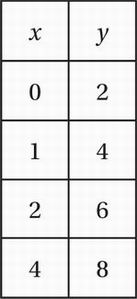# SAT Math Multiple Choice Question 693: Answer and Explanation

### Test Information

Question: 693

3.Based on the ordered pairs in the table above, which of the following could express a relationship between x and y?

• A. y = x + 4
• B. y = 2x
• C. y = 2x + 2
• D. y = 2x + 4